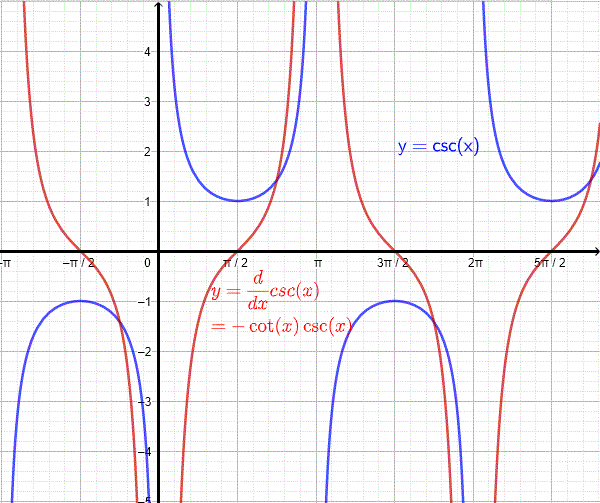# Proof of the Derivative of csc x

The proof of the calculation of the derivative of $\csc (x)$ is presented using the quotient rule of derivatives.

## Proof of the Derivative of csc x

A trigonometric identity relating $\csc x$ and $\sin x$ is given by $\csc x = \dfrac { 1 }{ \sin x }$ Use of the quotient rule of differentiation to find the derivative of $\csc x$; hence
$\displaystyle { \dfrac {d}{dx} \csc x = \dfrac {d}{dx} (\dfrac{ 1 }{\sin x}) = \dfrac { (\dfrac {d}{dx}1) { \sin x } - 1 (\dfrac {d}{dx} \sin x) } {\sin^2 x} }$

The derivative of 1 is equal to zero. Use the formulae for the
derivative of the trigonometric functions $\sin x$ given by $\dfrac {d}{dx}\sin x = \cos x$ and substitute to obtain

$\displaystyle {\dfrac {d}{dx} \csc x = \dfrac{ (0 - (\cos x) )}{\sin^2 x}}$

Simplify

$\displaystyle {= \dfrac{ - \cos x } {\sin^2 x} = - \dfrac{ \cos x }{\sin x} \dfrac{ 1 }{\sin x} = - \cot x \csc x}$

conclusion
$\displaystyle {\dfrac {d}{dx} \csc x = - \cot x \; \csc x}$

## Graph of csc x and its Derivative

The graphs of $\csc(x)$ and its derivative are shown below.## Derivative of the Composite Function csc (u(x))

Let us consider the composite function csc of another function u(x). Use the chain rule of differentiation to write

$\displaystyle \dfrac{d}{dx} \csc (u(x)) = (\dfrac{d}{du} \csc u) (\dfrac{d}{dx} u )$

Simplify

$= - \cot u \csc u \dfrac{d}{dx} u$

Conclusion

$\displaystyle \dfrac{d}{dx} \csc (u(x)) = - \cot u \; \csc u \; \dfrac{d}{dx} u$

Example 1
Find the derivative of the composite csc functions

1. $f(x) = \csc (-x^3+3)$
2. $g(x) = \csc (\cos(x))$
3. $h(x) = \csc (\dfrac{1}{x^2+1})$

Solution to Example 1

1. Let $u(x) = -x^3+3$ and therefore $\dfrac{d}{dx} u = \dfrac{d}{dx} (-x^3+3) = -3x^2$ and apply the rule for the composite csc function given above

$\displaystyle \dfrac{d}{dx} f(x) = - \cot u \csc u \dfrac{d}{dx} u = - \cot (-x^3+3) \csc (-x^3+3) \times (-3x^2)$

$= 3x^2 \; \cot (-x^3+3) \; \csc (-x^3+3)$

2. Let $u(x) = \cos x$ and therefore $\dfrac{d}{dx} u = \dfrac{d}{dx} \cos x = - \sin x$ and apply the above rule of differentiation for the composite csc function

$\displaystyle \dfrac{d}{dx} g(x) = - \cot u \csc u \dfrac{d}{dx} u = - \cot (\cos x) \csc (\cos x) \times (- \sin x)$

$= \sin x \;\cot (\cos x) \; \csc (\cos x)$

3. Let $u(x) = \dfrac{1}{x^2+1}$ and therefore $\dfrac{d}{dx} u = -\dfrac{2x}{(x^2+1)^2}$ and apply the rule of differentiation for the composite csc function obtained above

$\displaystyle \dfrac{d}{dx} h(x) = - \cot u \csc u \dfrac{d}{dx} u = - \cot (\dfrac{1}{x^2+1}) \csc (\dfrac{1}{x^2+1}) \times (-\dfrac{2x}{(x^2+1)^2})$

$= \dfrac{2x}{(x^2+1)^2} \; \cot (\dfrac{1}{x^2+1}) \;\csc (\dfrac{1}{x^2+1})$